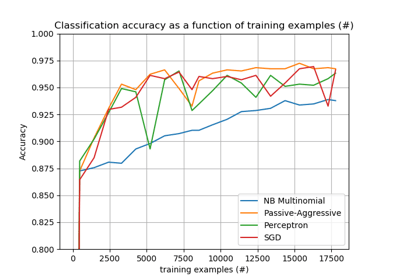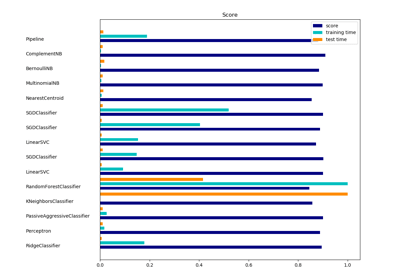/scikit-learn

# sklearn.feature_extraction.text.HashingVectorizer

`class sklearn.feature_extraction.text.HashingVectorizer(input=’content’, encoding=’utf-8’, decode_error=’strict’, strip_accents=None, lowercase=True, preprocessor=None, tokenizer=None, stop_words=None, token_pattern=’(?u)\b\w\w+\b’, ngram_range=(1, 1), analyzer=’word’, n_features=1048576, binary=False, norm=’l2’, alternate_sign=True, non_negative=False, dtype=<class ‘numpy.float64’>)` [source]

Convert a collection of text documents to a matrix of token occurrences

It turns a collection of text documents into a scipy.sparse matrix holding token occurrence counts (or binary occurrence information), possibly normalized as token frequencies if norm=’l1’ or projected on the euclidean unit sphere if norm=’l2’.

This text vectorizer implementation uses the hashing trick to find the token string name to feature integer index mapping.

• it is very low memory scalable to large datasets as there is no need to store a vocabulary dictionary in memory
• it is fast to pickle and un-pickle as it holds no state besides the constructor parameters
• it can be used in a streaming (partial fit) or parallel pipeline as there is no state computed during fit.

There are also a couple of cons (vs using a CountVectorizer with an in-memory vocabulary):

• there is no way to compute the inverse transform (from feature indices to string feature names) which can be a problem when trying to introspect which features are most important to a model.
• there can be collisions: distinct tokens can be mapped to the same feature index. However in practice this is rarely an issue if n_features is large enough (e.g. 2 ** 18 for text classification problems).
• no IDF weighting as this would render the transformer stateful.

The hash function employed is the signed 32-bit version of Murmurhash3.

Read more in the User Guide.

Parameters: `input : string {‘filename’, ‘file’, ‘content’}` If ‘filename’, the sequence passed as an argument to fit is expected to be a list of filenames that need reading to fetch the raw content to analyze. If ‘file’, the sequence items must have a ‘read’ method (file-like object) that is called to fetch the bytes in memory. Otherwise the input is expected to be the sequence strings or bytes items are expected to be analyzed directly. `encoding : string, default=’utf-8’` If bytes or files are given to analyze, this encoding is used to decode. `decode_error : {‘strict’, ‘ignore’, ‘replace’}` Instruction on what to do if a byte sequence is given to analyze that contains characters not of the given `encoding`. By default, it is ‘strict’, meaning that a UnicodeDecodeError will be raised. Other values are ‘ignore’ and ‘replace’. `strip_accents : {‘ascii’, ‘unicode’, None}` Remove accents and perform other character normalization during the preprocessing step. ‘ascii’ is a fast method that only works on characters that have an direct ASCII mapping. ‘unicode’ is a slightly slower method that works on any characters. None (default) does nothing. Both ‘ascii’ and ‘unicode’ use NFKD normalization from `unicodedata.normalize`. `lowercase : boolean, default=True` Convert all characters to lowercase before tokenizing. `preprocessor : callable or None (default)` Override the preprocessing (string transformation) stage while preserving the tokenizing and n-grams generation steps. `tokenizer : callable or None (default)` Override the string tokenization step while preserving the preprocessing and n-grams generation steps. Only applies if `analyzer == 'word'`. `stop_words : string {‘english’}, list, or None (default)` If ‘english’, a built-in stop word list for English is used. There are several known issues with ‘english’ and you should consider an alternative (see Using stop words). If a list, that list is assumed to contain stop words, all of which will be removed from the resulting tokens. Only applies if `analyzer == 'word'`. `token_pattern : string` Regular expression denoting what constitutes a “token”, only used if `analyzer == 'word'`. The default regexp selects tokens of 2 or more alphanumeric characters (punctuation is completely ignored and always treated as a token separator). `ngram_range : tuple (min_n, max_n), default=(1, 1)` The lower and upper boundary of the range of n-values for different n-grams to be extracted. All values of n such that min_n <= n <= max_n will be used. `analyzer : string, {‘word’, ‘char’, ‘char_wb’} or callable` Whether the feature should be made of word or character n-grams. Option ‘char_wb’ creates character n-grams only from text inside word boundaries; n-grams at the edges of words are padded with space. If a callable is passed it is used to extract the sequence of features out of the raw, unprocessed input. `n_features : integer, default=(2 ** 20)` The number of features (columns) in the output matrices. Small numbers of features are likely to cause hash collisions, but large numbers will cause larger coefficient dimensions in linear learners. `binary : boolean, default=False.` If True, all non zero counts are set to 1. This is useful for discrete probabilistic models that model binary events rather than integer counts. `norm : ‘l1’, ‘l2’ or None, optional` Norm used to normalize term vectors. None for no normalization. `alternate_sign : boolean, optional, default True` When True, an alternating sign is added to the features as to approximately conserve the inner product in the hashed space even for small n_features. This approach is similar to sparse random projection. New in version 0.19. `non_negative : boolean, optional, default False` When True, an absolute value is applied to the features matrix prior to returning it. When used in conjunction with alternate_sign=True, this significantly reduces the inner product preservation property. Deprecated since version 0.19: This option will be removed in 0.21. `dtype : type, optional` Type of the matrix returned by fit_transform() or transform().

#### Examples

```>>> from sklearn.feature_extraction.text import HashingVectorizer
>>> corpus = [
...     'This is the first document.',
...     'This document is the second document.',
...     'And this is the third one.',
...     'Is this the first document?',
... ]
>>> vectorizer = HashingVectorizer(n_features=2**4)
>>> X = vectorizer.fit_transform(corpus)
>>> print(X.shape)
(4, 16)
```

#### Methods

 `build_analyzer`() Return a callable that handles preprocessing and tokenization `build_preprocessor`() Return a function to preprocess the text before tokenization `build_tokenizer`() Return a function that splits a string into a sequence of tokens `decode`(doc) Decode the input into a string of unicode symbols `fit`(X[, y]) Does nothing: this transformer is stateless. `fit_transform`(X[, y]) Transform a sequence of documents to a document-term matrix. `get_params`([deep]) Get parameters for this estimator. `get_stop_words`() Build or fetch the effective stop words list `partial_fit`(X[, y]) Does nothing: this transformer is stateless. `set_params`(**params) Set the parameters of this estimator. `transform`(X) Transform a sequence of documents to a document-term matrix.
`__init__(input=’content’, encoding=’utf-8’, decode_error=’strict’, strip_accents=None, lowercase=True, preprocessor=None, tokenizer=None, stop_words=None, token_pattern=’(?u)\b\w\w+\b’, ngram_range=(1, 1), analyzer=’word’, n_features=1048576, binary=False, norm=’l2’, alternate_sign=True, non_negative=False, dtype=<class ‘numpy.float64’>)` [source]
`build_analyzer()` [source]

Return a callable that handles preprocessing and tokenization

`build_preprocessor()` [source]

Return a function to preprocess the text before tokenization

`build_tokenizer()` [source]

Return a function that splits a string into a sequence of tokens

`decode(doc)` [source]

Decode the input into a string of unicode symbols

The decoding strategy depends on the vectorizer parameters.

Parameters: `doc : string` The string to decode
`fit(X, y=None)` [source]

Does nothing: this transformer is stateless.

Parameters: `X : array-like, shape [n_samples, n_features]` Training data.
`fit_transform(X, y=None)` [source]

Transform a sequence of documents to a document-term matrix.

Parameters: `X : iterable over raw text documents, length = n_samples` Samples. Each sample must be a text document (either bytes or unicode strings, file name or file object depending on the constructor argument) which will be tokenized and hashed. `y : any` Ignored. This parameter exists only for compatibility with sklearn.pipeline.Pipeline. `X : scipy.sparse matrix, shape = (n_samples, self.n_features)` Document-term matrix.
`get_params(deep=True)` [source]

Get parameters for this estimator.

Parameters: `deep : boolean, optional` If True, will return the parameters for this estimator and contained subobjects that are estimators. `params : mapping of string to any` Parameter names mapped to their values.
`get_stop_words()` [source]

Build or fetch the effective stop words list

`partial_fit(X, y=None)` [source]

Does nothing: this transformer is stateless.

This method is just there to mark the fact that this transformer can work in a streaming setup.

Parameters: `X : array-like, shape [n_samples, n_features]` Training data.
`set_params(**params)` [source]

Set the parameters of this estimator.

The method works on simple estimators as well as on nested objects (such as pipelines). The latter have parameters of the form `<component>__<parameter>` so that it’s possible to update each component of a nested object.

Returns: self
`transform(X)` [source]

Transform a sequence of documents to a document-term matrix.

Parameters: `X : iterable over raw text documents, length = n_samples` Samples. Each sample must be a text document (either bytes or unicode strings, file name or file object depending on the constructor argument) which will be tokenized and hashed. `X : scipy.sparse matrix, shape = (n_samples, self.n_features)` Document-term matrix.

## Examples using `sklearn.feature_extraction.text.HashingVectorizer`Out-of-core classification of text documentsClustering text documents using k-meansClassification of text documents using sparse features